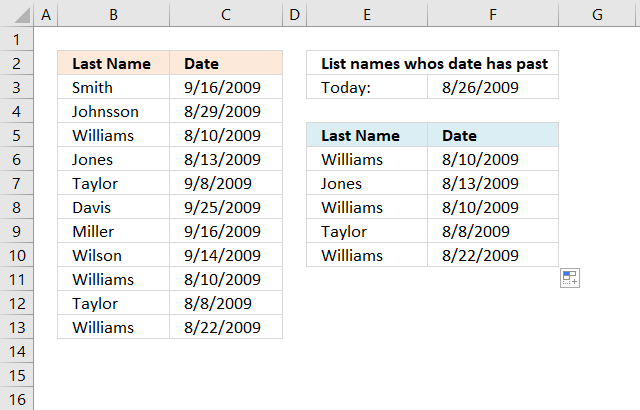Author: Oscar Cronquist Article last updated on November 29, 2018Question: I have a column "B" with a last name.. I have another columb with a date in it "C"... I need to be able to list the names from columb B on a second sheet whos column c date has past....

Array formula in E6:

=INDEX(\$B\$3:\$B\$13, SMALL(IF(\$C\$3:\$C\$13<\$F\$3, ROW(\$C\$3:\$C\$13)-MIN(ROW(\$C\$3:\$C\$13))+1, ""), ROWS(\$F\$6:F6)))

Formula in F6:

=INDEX(\$C\$3:\$C\$13, SMALL(IF(COUNTIF(E6, \$B\$3:\$B\$13)*(\$C\$3:\$C\$13<\$F\$3), MATCH(ROW(\$B\$3:\$B\$13), ROW(\$B\$3:\$B\$13)), ""), COUNTIF(\$E\$6:E6, E6)))

To enter an array formula, type the formula in a cell then press and hold CTRL + SHIFT simultaneously, now press Enter once. Release all keys.

The formula bar now shows the formula with a beginning and ending curly bracket telling you that you entered the formula successfully. Don't enter the curly brackets yourself.

### Explaining formula in cell E6

#### Step 1 - Find rows in cell range with date past

Dates in Excel are numbers formatted as dates. 1/1/1900 is 1. 1/1/2000 is 36526. If you enter a date in a cell Excel automatically converts and formats the date, however, if you enter a date in a formula you need to use the DATE function to convert the date to an Excel date.

\$C\$3:\$C\$13<\$F\$3

becomes

{40072; 40054; 40035; 40038; 40064; 40081; 40072; 40070; 40035; 40033; 40047}<40051

and returns

{FALSE; FALSE; TRUE; TRUE; FALSE; FALSE; FALSE; FALSE; TRUE; TRUE; TRUE}.

#### Step 2 - Convert TRUE to corresponding row number

The following IF function returns the row number if date number is less than number in cell F3. FALSE returns "" (nothing).

IF(\$C\$3:\$C\$13<\$F\$3,ROW(\$C\$3:\$C\$13)-MIN(ROW(\$C\$3:\$C\$13))+1,"")

becomes

IF({FALSE; FALSE; TRUE; TRUE; FALSE; FALSE; FALSE; FALSE; TRUE; TRUE; TRUE},{1;2;3;4;5;6;7;8;9;10;11},"")

and returns

{"";"";3; 4;"";"";""; "";9;10; 11}

#### Step 3 - Extract k-th smallest row number

The SMALL function makes sure that a new value is returned in each row, the second argument \$F\$6:F6 is expanding when you copy the cell and paste to cells below. This adds 1 to the second argument for each cell below.

SMALL(IF(\$C\$3:\$C\$13<\$F\$3, ROW(\$C\$3:\$C\$13)-MIN(ROW(\$C\$3:\$C\$13))+1, ""), ROWS(\$F\$6:F6))

becomes

SMALL({"";"";3;4;""; "";"";"";9;10;11}, ROWS(\$F\$6:F6))

becomes

SMALL({"";"";3;4; "";"";"";"";9; 10;11}, 1)

and returns 3.

#### Step 4 - Return value

The INDEX function returns a value based on a row and column number. Our cell range is only one column so we need only the row number to get the correct value.

INDEX(\$B\$3:\$B\$13,SMALL(IF(\$C\$3:\$C\$13<\$F\$3,ROW(\$C\$3:\$C\$13)-MIN(ROW(\$C\$3:\$C\$13))+1,""),ROWS(\$F\$6:F6)))

becomes

INDEX(\$B\$3:\$B\$13,3)

becomes

INDEX({"Smith"; "Johnsson"; "Williams"; "Jones"; "Taylor"; "Davis"; "Miller"; "Wilson"; "Williams"; "Taylor"; "Williams"},3)

and returns "Williams" in cell E6.

### Explaining formula in cell F6

#### Step 1 - Find adjacent value in cell range

COUNTIF(E6,\$B\$3:\$B\$13)

returns

{0;0;1;0;0;0;0;0;1;0;1}

#### Step 2 - Find rows with date past today

(\$C\$3:\$C\$13<\$F\$3)

returns

{FALSE; FALSE; TRUE; TRUE; FALSE; FALSE; FALSE; FALSE; TRUE; TRUE; TRUE}

#### Step 3 - Multiply arrays

COUNTIF(E6,\$B\$3:\$B\$13)*(\$C\$3:\$C\$13<\$F\$3)

becomes

{0;0;1;0;0;0;0;0;1;0;1}*{FALSE; FALSE; TRUE; TRUE; FALSE; FALSE; FALSE; FALSE; TRUE; TRUE; TRUE}

and returns

{0;0;1;0;0;0;0;0;1;0;1}.

#### Step 4 - Replace TRUE with corresponding row number

IF(COUNTIF(E6,\$B\$3:\$B\$13)*(\$C\$3:\$C\$13<\$F\$3), MATCH(ROW(\$B\$3:\$B\$13), ROW(\$B\$3:\$B\$13)),"")

becomes

IF({0;0;1;0;0;0;0;0;1;0;1}, MATCH(ROW(\$B\$3:\$B\$13), ROW(\$B\$3:\$B\$13)),"")

becomes

IF({0;0;1;0;0;0;0;0;1;0;1}, {1; 2; 3; 4; 5; 6; 7; 8; 9; 10; 11},"")

and returns

{"";"";3;"";"";"";"";"";9;"";11}.

#### Step 5 - Extract correct row number

The second argument in the SMALL function contains the COUNTIF function, it is designed to count how many times the adjacent value has been displayed before. The first cell reference in the COUNTIF function expands as the cell is copied to cells below. This lets the formula keep track of previous values, we want it to return the correct date value even if there are duplicates in column B.

SMALL(IF(COUNTIF(E6, \$B\$3:\$B\$13)*(\$C\$3:\$C\$13<\$F\$3), MATCH(ROW(\$B\$3:\$B\$13), ROW(\$B\$3:\$B\$13)), ""), COUNTIF(\$E\$6:E6,E6))

becomes

SMALL({"";"";3;"";"";"";"";"";9;"";11},  COUNTIF(\$E\$6:E6,E6))

becomes

SMALL({"";"";3;"";"";"";"";"";9;"";11},  1)

and returns 3.

#### Step 6 - Return date value

INDEX(\$C\$3:\$C\$13, SMALL(IF(COUNTIF(E6, \$B\$3:\$B\$13)*(\$C\$3:\$C\$13<\$F\$3), MATCH(ROW(\$B\$3:\$B\$13), ROW(\$B\$3:\$B\$13)), ""), COUNTIF(\$E\$6:E6, E6)))

becomes

INDEX(\$C\$3:\$C\$13, 3)

and returns "8/10/2009" in cell F6.